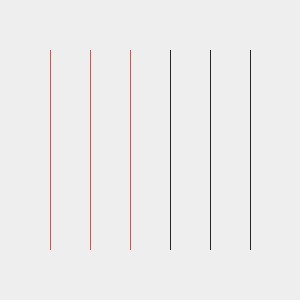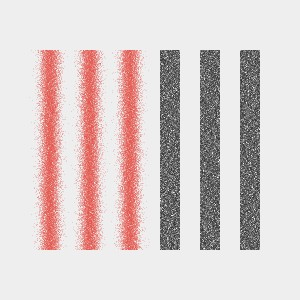randomGaussian()

`randomGaussian()` is a function that gives you a random number from a normal (0,1) distribution. This means that the average of all returned numbers is `0` and that almost all values are between `(-3,3)`. Actually you could get any value but values outside of the `(-3,3)` range will be rare.

How to use it

• `float val = randomGaussian();` - without any special parameters, the function will return some floating point number, with a very high probability of hitting the `(-3,3)` range
• `float val = 10.0 * randomGaussian();` - to scale your result, simply multiply by the desired amount; in this case the returned value will very probably be in the `(-30,30)` range
• `float val = 5.0 + 10.0 * randomGaussian();` - to shift the range of probable numbers just add the desired offset; in this case the returned value will very probably be in the `(-25,35)` range

What is it for

Generally it's primarily used to distort rendered points or to blur things.

Example: lines

Let's start with rendering lines. But we will not simply use the `line()` function; instead we will draw them point by point.

``````color black = color(34, 34, 34); // #222222
color white = color(238, 238, 238); // #EEEEEE
color red = color(225, 76, 69); // #E14C45

void setup() {
size(300, 300);
smooth(8);
noFill();
strokeWeight(0.8);
background(white);

for (int x=50; x<=250; x+=40) {
for (float y=50; y<250; y+=0.01) {

if (x<150) {
stroke(red, 100);
point(x,y);
} else {
stroke(black, 100);
point(x,y);
}

}
}
}
``````Now let's distort the red points with `randomGaussian()` and the black points with regular `random()` to illustrate the difference between the two functions.

``````color black = color(34, 34, 34); // #222222
color white = color(238, 238, 238); // #EEEEEE
color red = color(225, 76, 69); // #E14C45

void setup() {
size(300, 300);
smooth(8);
noFill();
strokeWeight(0.8);
background(white);

for (int x=50; x<=250; x+=40) {
for (float y=50; y<250; y+=0.01) {

if (x<150) {
stroke(red, 100);
point(x+5*randomGaussian(), y); // add some random value to the x position
} else {
stroke(black, 100);
point(x+5*random(-2, 2), y); // add some random value to the x position
}

}
}
}
``````You can easily see the difference now. The pixels of the red bars are concentrated near the middle while the pixels of the black bars are uniformly distributed.

``````color black = color(34, 34, 34); // #222222
color white = color(238, 238, 238); // #EEEEEE
color red = color(225, 76, 69); // #E14C45

void setup() {
size(300, 300);
smooth(8);
noFill();
strokeWeight(0.8);
background(white);

for (int x=50; x<=250; x+=40) {
for (float y=50; y<250; y+=0.01) {

// transform y to angle from -PI, PI
float sy = map(y, 50, 250, -PI, PI);
// transform angle to values from -1 through 1 to -1
float csy = cos(sy);
// transform above to go from 0 to 5 and back to 0 (smoothly)
float f = map(csy, -1, 1, 0, 5);

if(x<150) {
stroke(red, 100);
point(x+f*randomGaussian(), y);
} else {
stroke(black, 100);
point(x+f*random(-2,2), y);
}
}
}
}
``````Example: Blurring square

In the following example we'll render a square from points and use `randomGaussian()` to spread the points on each frame. `float s = 10*sin(step);` this statement calculates the factor the value returned by randomGaussion() will be multiplied with. in this case `s` will sway between `-10` and `10`.

``````color black = color(34, 34, 34); // #222222
color white = color(238, 238, 238); // #EEEEEE
color red = color(225, 76, 69); // #E14C45

void setup() {
size(300, 300);
smooth(8);
noFill();
stroke(red,40);
}

float step = 0;
void draw() {
background(white);
translate(150, 150); // set origin in the middle of the screen

float s = 10*sin(step);

for(float x=-40;x<40;x+=0.3) {
for(float y=-40;y<40;y+=0.3) {
point(x+randomGaussian()*s,y+randomGaussian()*s);
}
}
step += PI/30.0;
}
``````